Document Type : Original Research Article

Authors

1 Department of Mathematics, Government First Grade College, Holalkere-577526, Karnataka, India

2 Department of Mathematics, Alliance School of Applied Mathematics, Alliance University, Bangalure-562106, Karnataka, India

3 Department of Mathematics, Bapuji Institute of Engineering & Technology, Davanagere-577004, India

4 Department of Mathematics, School of Engineering, Dayananda Sagar University, Bangalure-560078, India

Abstract

The fundamental topology of the structure of chemical compounds can be better understood by the method of topological indices /numerical descriptors. Topological index depicts the chemical characteristic of a molecule in numerical form. Topological indices are used for modelling of physicochemical, biological, and pharmacokinetic properties of the compounds. It plays vital role in the QSAR/QSPR studies. Descriptor’s ability to extract information typically depends on the type of molecular representation used and the specified algorithm. These numerical values help the researchers in choosing the right compound for the drug design. Chitin and chitosan derivatives act as excellent suppressor of anti-tumour and anticancer activities in living beings. The increasing morbidity and mortality rate worldwide is correlated with two most important diseases viz., obesity and diabetes. To improve health condition and prevention of chronic diseases such as asthma, arthritis, hepatitis, gastritis, atherosclerosis etc, chitin, chitosan and their derivatives play as immune-enhancing anti-inflammatory potential. As chitin and chitosan have remarkable applications discussed above, this work pinpoints on computing a polynomial from which topological indices can be extracted for specific values of the parameters. In this work, the focus is on a type of polynomial known as M-polynomial from which various 11 degree-based TIs are derived for molecular graph of chitosan derivatives such as α, β and γ -chitins.

Graphical Abstract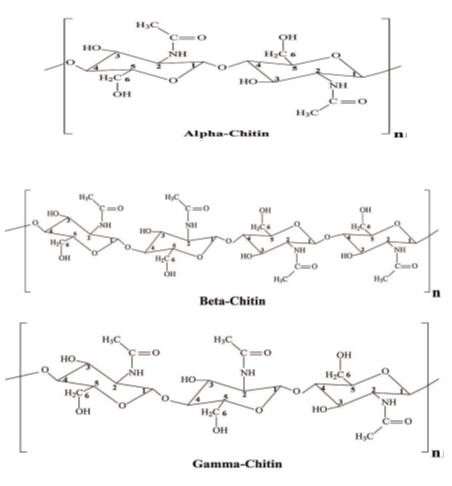Keywords

###### ##### Full Text

Introduction

The natural biopolymers that can be processed easily in different forms are chitin and chitosan. They can be formed into membranes, sponges, micro-particles, scaffolds used in wound healing, drug delivery, gene therapy, and tissue engineering. Chitin is rigid, hard and white natural mucobiopolymer that is the second most copious biopolymer on the earth. Carbon, hydrogen, and oxygen combine to form compound such as saccharides also known as carbohydrates.

The smallest carbohydrates are the monosaccharides which in turn become polysaccharides by the formation of building blocks. A short chain of monosaccharides refers to oligosaccharides, often produced by anabolic or catabolic process. Depending on the number of monosaccharides, it is classified as disaccharides having two monosaccharides, trisaccharide with three, tetra saccharides containing four, penta-saccharides having five and so on. Monosaccharides play a significant role in generating energy consumption. Their derivatives are found in important nucleic acids like DNA, ATP, RNA, and key components of coenzymes.

Carbohydrates in nonnumeric, oligomer, and polymeric form, plays crucial role in cell interactions, hormones, tumour metastasis, and antibody recognition. The deacetylation of chitin results in chitosan, which is non-toxic, biocompatible, and biodegradable [1, 2].

Chitin/chitosan show captivating physiological, biological, and pharmacological properties. Chitosan obtained from Porifera has multiple applications in the scaffolds of marine sponges. Because of its natural characteristics such as biocompatibility, biodegradability, non-toxicity, and humidity absorption, chitin and chitosan are suitable for various applications in many fields.

Biological activities and applications of chitin and chitosan derivatives

Various researchers vouch that chitin and chitosan are adaptable antimicrobials because of its intrinsic and extrinsic factors such as degree of polymerization, deacetylation, and effective dose in experimental methods. Chitosan imparts preferable antimicrobial activity than chitin due to its finer solubility property and free amine group. Most of the biological applications are possible due to the antimicrobial activity of chitin/chitosan and they are supported experimentally. The mechanism of antimicrobial suggested that the amino sugar may enter the cell and made complex with DNA by binding at low molecular weight constituents. It was also observed that DNA leads to the obstruction of transcription and translation process. To improve health condition and prevention of deep-rooted diseases like asthma, arthritis, hepatitis, gastritis, atherosclerosis etc., chitin, chitosan, and their derivatives play an immune-enhancing anti-inflammatory potential .

The study of these properties is witnessed by advocating them in animal models. The interactions of chitin/chitosan and their derivative products are reported to have significance in checking the bleeding by contracting tissues, platelet aggregation, and blood coagulation factors. The bleeding time is therapeutically proved to be brought to normal range in rabbit model when chitosan is used in the treatment.

One of the most common cardiovascular diseases is high blood pressure also known as hypertension. Based on the magnificent hemostatic activity, chitin and chitosan derivatives have been explored for over production of angiotensin II in which its enzyme plays a significant role. Supplementing chitosan normalizes the systolic blood pressure in human. The uncontrolled cell division because of environmental and physical factors leads to cancer. Chitin and chitosan derivatives act as excellent suppressor of anti-tumour and anticancer activities in living beings .

The increasing morbidity and mortality rate worldwide are correlated with two most important diseases such as obesity and diabetes.

Despite a lot of research in improving the diabetic medicine, there is a huge demand for the natural antidiabetic agents which cannot be considered as pharmaceutical agent. In this situation, chitin/chitosan derivatives show a reasonable antidiabetic and hypocholesteraemia activity directly or indirectly. Chitosan has been found effective against type 1 and type 2 diabetes. A natural cat-ionic polymer that is derived from chitin is chitosan. It has received greater focus in drug delivery mainly because of its biodegradable, biocompatible, and non-toxic nature. The drug release is controlled because of the presence of amine groups and hydroxyl. Majority of the relevance of graph theory in chemistry facilitates the study of many tools such as topological indices, polynomials, distance-based indices, eigen values, and so on. These tools provide treasure of data regarding a chemical compound that correlates with its structure. A topological index is a numerical value which defines the topology of the structure of the molecule and helps in predicting a range of molecular properties. It has widened its applications in many fields such as pharmacological medicine, toxicity, and theoretical chemistry [5-8]. The models of QSPR/QSAR/QSTR of chemical compounds are used to design a drug as it depends on properties (physicochemical) with high degree of exactness [9-12].

Initially, Harold Wiener in 1947 introduced the first topological index, Wiener index which is distance-based index. Since then, numerous studies have resulted in the evaluation of various TIs by many researchers. The numerical invariant defines a relation between the physical structure and the chemical properties of the compounds.

Wiener affirmed that the Wiener index suits best with the boiling point of the alkane by modelling the index on QSPR, following which it was used to link the structure to their respective biological activities and to interpret various properties of the compounds [13, 14]. The most popular and the oldest index in QSAR and QSPR models is the Randic index [15, 16]. Subsequently, Bollobas and Erdos introduced the generalised version of Randic index . An alternative of the Randic index was the Harmonic index, introduced by Fatlowicz.

The most accepted indices introduced in 1972 by Gutman and Trinajstic are the first and the second Zagreb indices .

They have been found very impressive in determining the total π -electron energy of molecules . Ranjini et al. redefined the Zagreb indices for a graph  as redefined the first, the second, and the third Zagreb indices. The augmented Zagreb index is found to be best in estimating the heat of formation of alkanes . The Forgotten index introduced by Gutman et al., is found to be almost identical in prediction performance to that of the original Zagreb index. Forgotten index was further studied by Furtula . Various physicochemical properties can be illustrated with a high degree of accuracy using neighbourhood degree sum-based TIs. Ghorbani et al. defined neighbourhood degree of vertices index named as third version of Zagreb index [22–23].

Later, it was learnt by Hosamani that there is a high association of neighbourhood-based indices with the entropy of octane isomers which resulted in the introduction of Sanskruti index . There were several neighbourhood-based indices introduced by many researchers globally which are in use in the study of QSAR/QSPR/QSTR analysis [25-27].

M-polynomial

There are many tools in graph theory that play an important role in various fields. Polynomials are one such tool which contribute to the applications of graph theory [29-31]. There are many polynomials introduced, in which Hosoya polynomial  played a significant role in distance-based polynomials. Recently, degree-based polynomial was introduced in 2015 , known as M-polynomial and subsequently NM-polynomial  was introduced which is neighbourhood degree-based polynomial. This article focuses on computation of M-polynomial through which eleven degree-based topological indices are derived for chitosan and their derivatives.

These indices are helpful in the studies of theoretical chemistry, pharmaceutical research, drug design, and other fields. Because of its broad applications, M-polynomial is used to obtain the formulae for topological indices that are degree-based and play a key role to appreciate various properties of a molecular structure. It is always preferable to find a compressed way to obtain various TIs using a single polynomial. This results in the usage of M-polynomial [34, 35]. These polynomials help in the study and investigation of properties using topological indices (TIs). The prediction of characteristics of novel drugs depends on the features of a molecular compound through its molecular graph. Consider a molecular graph G = (V, E), where V (G) denotes vertex set and E (G) denotes edge set. The common representations such as u V (G) is a vertex and d(u) are the degree of the vertex u, denotes the number of edges incident to the vertex u [36-38].

Definition 1.

The M-polynomial for a graph G is defined as follow: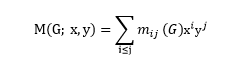such that mij , where i, j ≥ 1 denotes the cardinality of edges uv ∈ E(G), where {d(u), d(v)}={i, j}. Here, d (u), d(v) indicates number of edges incident to the vertices u and v in G. The list of TIs derived from M-polynomial are presented in Table.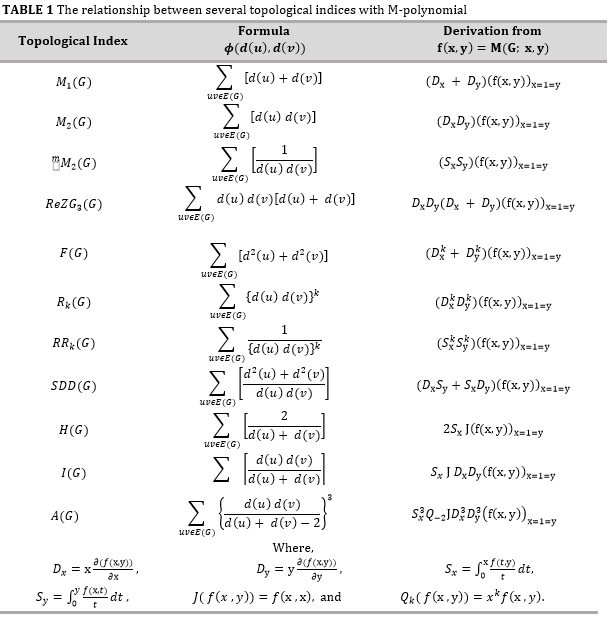Results and discussion

In this article, M-polynomial approach is employed to compute TIs for the derivatives of chitosan (Figure 1). Simple graphs are modelled for the molecular structure of chitosan derivatives viz., α, β, and γ-chitins. The tools used for computing these results comprise of vertex and edge partition along with combinatorial methods .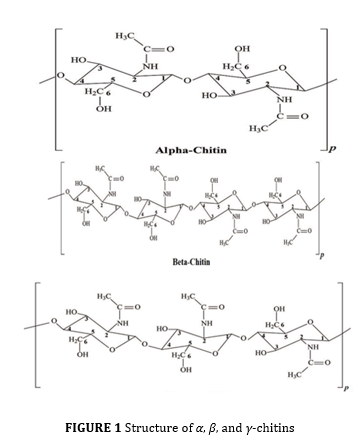The significance of the study

Chitosan is the most abundant polysaccharide available after cellulose. It is a very useful compound that has found its applications in various fields because of its interesting properties. It plays a significant role in the drug delivery, food, textile, pharmacy, waste management, and diode. It has marvellous properties like healing, antimicrobial, and antifungal which are significant in drug making. Hydro gels formed by chitosan have attracted lots of attention in the pharmaceutical field in the usage of lysozyme and fabrication of dressings.

It will suck wound secretions and govern the hydration of the wounded region. Chitosan derivatives are proven to be the safest choice in the applications of biomaterials that are extensively used for medical purposes. It is evident that there is a large volume of studies taking place on polysaccharide [40, 41] gradable, polymer membranes, and nano-fibres in the medical field. A class of materials that are integrated from chitosan and its derivatives are hydrogels used in producing contact lenses, wound dressings, and hygiene products. The significant characteristics and natural abundance of chitosan (hydrogels) like malleability, non-toxicity, and biocompatibility have attracted lot of studies from academia and industry .

M-polynomial of α-chitin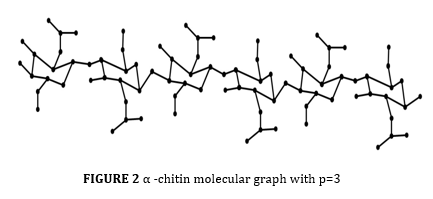Theorem 1

The M-polynomial for α-chitin is

M(αC; x, y) = (2p)xy2 + (6p + 2)xy3 + (14p − 2)x2y3 + (8p)x3y3.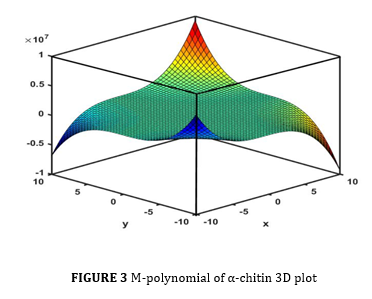Proof

Based on the Figure 2, it is observed that  and . Also, the edge set of αC is classified into four edge partitions depending on the vertex degrees are given as follow:

E12 = {uv E(αC)|d(u) = 1, d(v) = 2},

E13 = {uv E(αC)|d(u) = 1, d(v) = 3},

E23 = {uv E(αC)|d(u) = 2, d(v) = 3},

E33 = {uv E(αC)|d(u) = 3, d(v) = 3},

such that

m12 = 2p, m13 = 6p + 2, m23 = 14p − 2, and m33 = 8p.

Using the definition 1,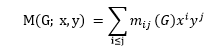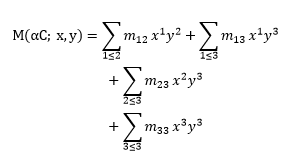= (2p)xy2 + (6p + 2)xy3 + (14p − 2)x2y3 + (8p)x3y3.

TIs of α-chitin are computed using Theorem 1 and Table 1, and is as follow:

Proposition 1

M1(αC) = 148p − 2.

M2(αC) = 178p − 6.

mM2(αC) = 6.222p + 0.333.

ReZG3(αC) = 936p − 36.

F(αC) = 396p – 6

Rk(αC) = 2k(2p) +3k(6p + 2) + 6k(14p − 2) + (8p).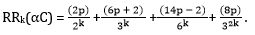SDD(αC) = 71.332p + 2.333.

H(αC) = 12.6p + 0.2.

I(αC) = 34.633p − 0.9.

A(αC) = 239.375p − 9.25.

Proof

Let f(x, y) = M(αC; x, y) = (2p)xy2 + (6p + 2)xy3 + (14p − 2)x2y3 + (8p)x3y3.

Then,

Dx f(x, y) = (2p)x1y2 +(6p + 2)x1y3 + 2(14p − 2)x2y3+ 3(8p)x3y3,

Dy f(x, y) = 2(2p) x1y2+ 3(6p + 2) x1y3+ 3(14p − 2) x2y3+ 3(8p) x3y3,

(Dx +Dy) f(x, y) = 3(2p) x1y2 + 4(6p + 2) x1y3+ 5(14p − 2) x2y3+ 6(8p) x3y3,

DyDxf(x, y) = 2(2p) x1y2+ 3(6p + 2) x1y3+ 6(14p − 2) x2y3+ 9(8p) x3y3,

(D2x + D2y) f(x, y) =5(2p) x1y2 + 10(6p + 2) x1y3+ 13(14p − 2) x2y3+ 18(8p) x3y3,

DkxDkyf(x, y) = 2k (2p) x1y2+ 3k (6p + 2) x1y3+ 6k (14p − 2) x2y3+  (8p) x3y3,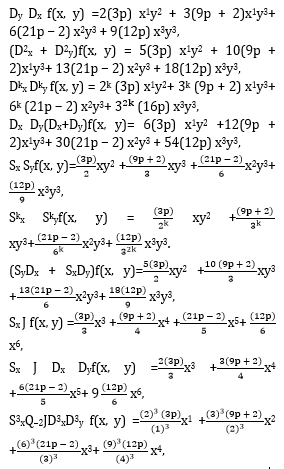According to Table 1, the following results are obtained:

M1(αC) = (Dx +Dy)f(x, y) |x=1=y = 148p − 2.

M2(αC) = (DxDy) f(x, y)| x=1=y= 178p − 6.

mM2(αC) = (SxSy)f (x, y)| x=1=y= 6.222p + 0.333.

ReZG3(αC) = DxDy(Dx + Dy) f(x, y)| x=1=y= 936p − 36.

F(αC) = (D2x + D2y) f(x, y)| x=1=y= 396p − 6.

Rk(αC) = (DkxDky) f(x, y)| x=1=y= 2k (2p) + 3k (6p + 2) + 6k (14p − 2) + 32k (8p).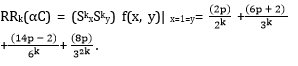SDD(αC) = (SyDx + SxDy) f(x, y)| x=1=y= 71.332p + 2.333.

H(αC) = 2Sx J f(x, y)| x=1=y= 12.6p + 0.2.

I(αC) = Sx J Dx Dy f(x, y)| x=1=y= 34.633p − 0.9.

A(αC) = S3xQ− 2 J D3x D3y f(x, y)| x=1=y = 239.375p − 9.25.

M-polynomial of β-chitin (βC)Theorem 2

The M-polynomial for β-chitin is as follow:

M(βC; x, y) = (4p)xy2 + (12p + 2)xy3 + (28p – 2)x2y3 + (16p)x3y3.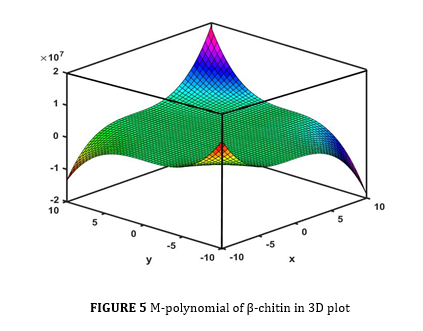Proof

The vertex and edge partitions of beta chitin from the Figure 4 are found to be |V (βC)| = 55p + 2 and |E(βC)| = 60p, respectively. The edge set of βC based on vertex degrees are given by

E12 = {uv ∈E(βC)|d(u) = 1, d(v) = 2},

E13 = {uv ∈E(βC)|d(u) = 1, d(v) = 3},

E23 = {uv ∈E(βC)|d(u) = 2, d(v) = 3},

E33 = {uv ∈E(βC)|d(u) = 3, d(v) = 3},

such that

m12 = 4p, m13 = 12p + 2, m23 = 28p − 2, and m33 = 16p.

Using definition 1,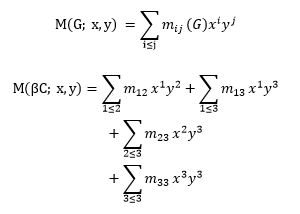= (4p)xy2 + (12p + 2)xy3 + (28p − 2)x2y3 + (16p)x3y3 .

From Theorem 2 and Table 1, various degree-based TIs of β-chitin are given by

Proposition 2.

M1(βC) = 296p − 2.

M2(βC) = 356p − 6.

mM2(βC) = 12.444p + 0.333.

ReZG3(βC) = 1872p − 36.

F(βC) = 792p − 6.

Rk(βC) = 2k(4p) + 3k(12p + 2) + 6k(28p − 2) + 32k(16p).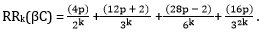SDD(βC) = 142.666p + 2.333.

H(βC) = 25.199p + 0.2.

I(βC) = 69.266p − 0.9.

A(βC) = 478.75p − 9.25.

Proof

Let f(x, y) = M (βC; x, y) = (4p) xy2 + (12p + 2)xy3 + (28p − 2)x2y3 + (16p)x3y3.

Then,

Dxf(x, y) = (4p)x1y2 + (12p + 2) x1y3+ 2(28p − 2)x2y3 + 3(16p)x3y3,

Dyf(x, y) = 2(4p) x1y2 +3(12p+2) x1y3+ 3(28p − 2) x2y3 + 3(16p) x3y3,

(Dx + Dy)f(x, y) = 3(4p) x1y2+ 4(12p + 2) x1y3+ 5(28p − 2) x2y3+ 6(16p) x3y3,

DyDxf(x, y) = 2(4p) x1y2+ 3(12p + 2) x1y3+ 6(28p − 2) x2y3+ 9(16p) x3y3,

(D2x + D2y)f(x, y) = 5(4p) x1y2+ 10(12p + 2) x1y3+ 13(28p − 2) x2y3+ 18(16p) x3y3,

DkxDkyf(x, y) = 2k (4p) x1y2+ 3k (12p + 2) x1y3+ 6k (28p − 2) x2y3+  (16p) x3y3,

DxDy(Dx+Dy)f(x, y) = 6(4p) x1y2+ 12(12p + 2) x1y3 + 30(28p − 2) x2y3+ 54(16p) x3y3,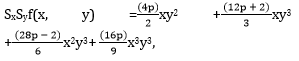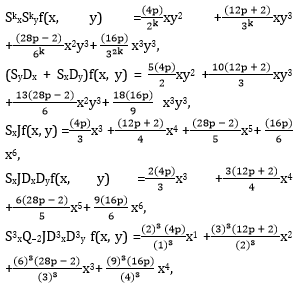Using Table 1, the following results are obtained as follow:

M1(βC) = (Dx + Dy)f(x, y) |x=1=y = 296p − 2.

M2(βC) = (DxDy)f(x, y)|x=1=y = 356p − 6.

mM2(βC) = (SxSy)f(x, y)|x=1=y = 12.444p + 0.333.

ReZG3(βC) = (DxDy) (Dx + Dy)f(x, y)|x=1=y = 31872p − 36.

F(βC) = (D2x + D2y)f(x, y)|x=1=y = 792p − 6.

Rk(βC) = (DkxDky)f(x, y)|x=1=y = 2k(4p) + 3k(12p + 2) + 6k(28p − 2) + 32k(16p).

RRk(βC) = (SkxSky )f(x, y)| x=1=y = (4p)2k + (12p + 2)3k + (28p − 2) 6k + (16p) 32k .

SDD(βC) = (DxSy + SxDy)f(x, y)|x=1=y = 142.666p + 2.333.

H(βC) = 2SxJf(x, y)|x=y=1 = 25.199p + 0.2.

I(βC) = SxJDxDyf(x, y)|x=y=1 = 69.266p − 0.9.

A(βC) = S3xQ-2JD3xD3y f(x, y)|x=y=1 = 478.75p –9.

M-polynomial of γ-chitin(γC)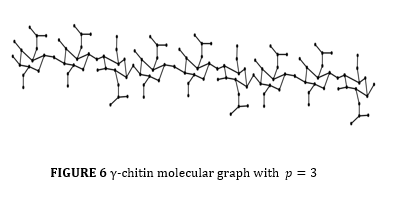Theorem 3

The M-polynomial for γ-chitin is:

M(γC; x, y) = (3p)xy2 + (9p + 2)xy3 + (21p − 2)x2y3+(12p)x3 y3.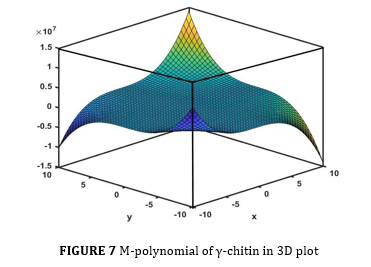Proof

Based the Figure 6, it is observed that |V (γC)| = 28p+1 and |E(γC)| = 30p. Also, the edge set of γC is classified into four edge partitions depending on the vertex degrees are given as follow:

E12 = {uv ∈E(γC)|d(u) = 1, d(v) = 2},

E13 = {uv ∈E(γC)|d(u) = 1, d(v) = 3},

E23 = {uv ∈E(γC)|d(u) = 2, d(v) = 3},

E33 = {uv ∈E(γC)|d(u) = 3, d(v) = 3},

such that

m12 = 3p, m13 = 9p + 2, m23 = 21p − 2, and m33 = 12p.

Using definition 1,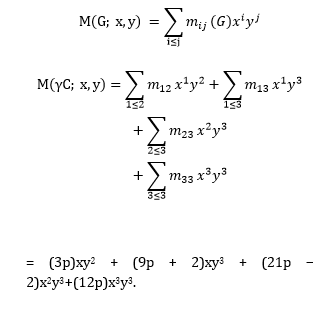Using Theorem 3 and Table 1, various degree-based TIs of the γ-chitin are given as follow:

Proposition 3

M1(γC) = 222p − 2.

M2(γC) = 267p − 6.

mM2(γC) = 9.333p + 0.333.

ReZG3(γC) = 1404p − 36.

F(γC) = 594p − 6.

Rk(γC) = 2k(3p) + 3k(9p + 2) + 6k(21p − 2) + (12p).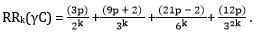SDD(γC) = 106.998p + 2.333.

H(γC) = 18.899p + 0.2.

I(γC) = 51.95p − 0.9.

A(γC) = 359.062p − 9.25.

Proof

Let f(x, y) = (3p)xy2 + (9p + 2)xy3 + (21p − 2) x2y3 + (12p)x3y3.

Then,

Dx f(x, y) = (3p) x1y2 + (9p + 2)x1y3+ 2(21p − 2) x2y3 + 3(12p) x3y3,

Dy f(x, y) = 2(3p) x1y2 + 3(9p + 2)x1y3+ 3(21p − 2) x2y3 + 3(12p) x3y3,

(Dx + Dy) f(x, y) = 3(3p) x1y2 + 4(9p + 2)x1y3+ 5(21p − 2) x2y3 + 6(12p) x3y3,

Dy Dx f(x, y) =2(3p) x1y2 + 3(9p + 2)x1y3+ 6(21p − 2) x2y3 + 9(12p) x3y3,

(D2x + D2y)f(x, y) = 5(3p) x1y2 + 10(9p + 2)x1y3+ 13(21p − 2) x2y3 + 18(12p) x3y3,

Dkx Dky f(x, y) = 2k (3p) x1y2+ 3k (9p + 2) x1y3+ 6k (21p − 2) x2y3+  (16p) x3y3,

Dx Dy(Dx+Dy)f(x, y)= 6(3p) x1y2 +12(9p + 2)x1y3+ 30(21p − 2) x2y3 + 54(12p) x3y3Using Table 1, we obtain the following results:

M1(γC)=(Dx + Dy)f(x, y)|x=1=y = 222p − 2.

M2(γC) = (Dx Dy)f(x, y)| x=1=y= 267p − 6.

mM2(γC) = SxSyf(x, y)| x=1=y = 9.333p + 0.333.

ReZG3(γC) = (Dx Dy) (Dx + Dy)f(x, y)| x=1=y = 1404p − 36.

F(γC) = (D2x + D2y)f(x, y)| x=1=y= 594p − 6.

Rk(γC) = (DkxDky)f(x, y)| x=1=y = 2k (3p) + 3k (9p + 2) + 6k (21p − 2) + 32k (12p).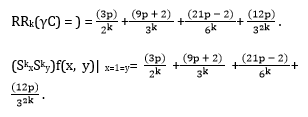SDD(γC) = (DxSy + SxDy)f(x, y)|x=1=y = 106.998p + 2.333.

H(γC) = 2SxJf(x, y)|x=y=1 = 18.899p + 0.2.

I(γC) = SxJDxDyf(x, y)|x=y=1 = 51.95p − 0.9.

A(γC) = S3xQ−2JD3xD3y f(x, y)|x=y=1 = 359.062p − 9.25

Comparison of indices using numerical data

Eleven topological indices are derived and computed for the chitosan derivatives and a detailed comparison is tabulated through Tables 2 to 4 for different values of n = 1 to 10. The TIs follow an upward trend as n increases.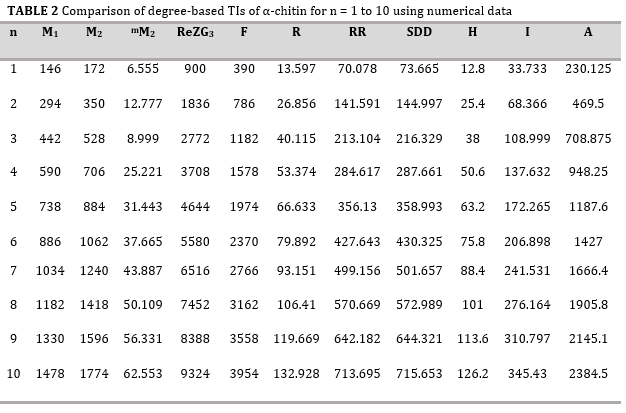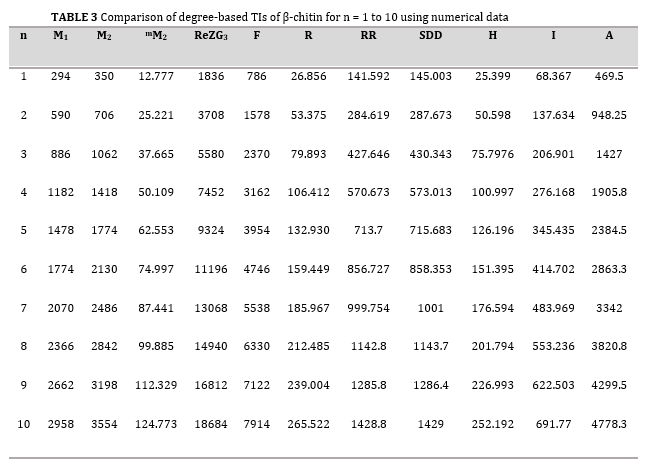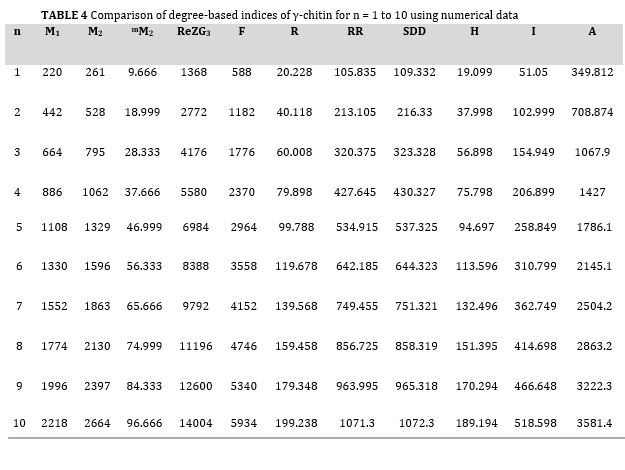Conclusion

A biodegradable polymer that is obtained from chitin is chitosan. It has varied applications in biomedical, textile, food storage, and many other fields. Hydrogels formed by chitosan are extensively used in medicines as they are absorbents of water-based liquids in the wounded areas. Chitosan is used as antimicrobial textiles for wound dressings. They are often used in drugs and proteins due to their inherent characteristics like non-toxicity, biocompatibility, hydrophilicity, etc. Chitosan finds its applications in technology as adsorbent of metals and dyes in waste-water from industrials wastes. This article focusses on finding M-polynomial of chitosan derivatives from which eleven degree-based TIs are computed. Thorough comparisons of the indices are tabulated. These polynomials are graphically represented in 3D plots (Figures 3, 5, and 7). The compounds used in drug discovery will be edible based on the range of topological indices. If the value of the index does not come under that range, it cannot be used as an excipient as it has to be consumed by the living beings. The compounds under this study include ample applications, and hence this study would be useful appropriately for the researchers who are willing to work on the same compounds.

Acknowledgements

Disclosure Statement

No potential conflict of interest was reported by the authors.

Funding

This research did not receive any specific grant from funding agencies in the public, commercial, or not-for-profit sectors.

Authors' contributions

All authors contributed to data analysis, drafting, and revising of the paper and agreed to be responsible for all the aspects of this work.

Orcid:

Shanmukha M.C.: https://orcid.org/0000-0002-9560-1209

Narendra V.H.: https://orcid.org/0009-0000-4976-2012

Mahalakshmi P.: https://orcid.org/0000-0001-6899-8354

Deepika T.: https://orcid.org/0000-0001-7908-1189

------------------------------------------------------------------------

How to cite this article: Narendra V.H., Usha A., Shanmukha M.C., Mahalakshmi P., Deepika T. Computing molecular descriptors of chitosan derivatives and its M-polynomial expressions. Journal of Medicinal and Pharmaceutical Chemistry Research, 2023, 5(10), 953-968. Link: https://www.echemcom.com/article_178534.html

------------------------------------------------------------------------

###### ##### References
 M.M Abo Elsoud, E.M. El Kady, Current trends in fungal biosynthesis of chitin and chitosan, Bull. Natl. Res. Cent., 2019, 43, 1-12. [Crossref], [Google Scholar], [Publisher]
 S.K. Halder, K.C. Mondal, Microbial valorization of chitinous bioresources for chitin extraction and production of chitooligomers and N-acetylglucosamine: trends, perspectives and prospects, Microbial biotechnology: Application in Food and Pharmacology, 2018, 2, 69-107. [Crossref], [Google Scholar], [Publisher]
 A. Anitha, S. Sowmya, P.S. Kumar, S. Deepthi, K.P Chennazhi, H. Ehrlich, M. Tsurkan, R. Jayakumar, Chitin and chitosan in selected biomedical applications, Prog. Polym. Sci., 2014, 39, 1644-1667. [Crossref], [Google Scholar], [Publisher]
 W. Wang, Q. Meng, Q. Li, J. Liu, M. Zhou, Z. Jin, K. Zhao, Chitosan derivatives and their application in biomedicine, Int. J. Mol. Sci., 2020, 21, 487. [Crossref], [Google Scholar], [Publisher]
 A. Aslam, M.K. Jamil, W. Gao, W. Nazeer, Topological aspects of some dendrimer structures, Nanotechnol Rev, 2018, 7, 123-129. [Crossref], [Google Scholar], [Publisher]
 I. Gutman, O.E. Polansky, Mathematical Concepts in Organic Chemistry, Springer Science & Business Media, 2012. [Google Scholar], [Publisher]
 N. Trinajstic, Chemical Graph Theory, 2nd revised CRC Press, Boca. Raton. FL, 1992. [Crossref], [Google Scholar], [Publisher]
 R. Todeschini, V. Consonni, Handbook of molecular descriptors, John Wiley & Sons, 2008. [Google Scholar], [Publisher]
 E. Estrada, L. Torres, L. Roriguez, I. Gutman, An atom-bond connectivity index: modelling the enthalpy of formation of alkanes, Indian J. Chem., 1998, 37, 849-855.  [Google Scholar], [Publisher]
 S.A.K. Kirmani, P. Ali, F. Azam, Topological indices and QSPR/QSAR analysis of some antiviral drugs being investigated for the treatment of COVID-19 patients, Int. J. Quantum Chem., 2021, 121, e26594. [Crossref], [Google Scholar], [Publisher]
 S. Hayat, M. Imran, J.B. Liu, Correlation between the Estrada index and π - electronic energies for benzenoid hydrocarbons with applications to boron nanotubes, Int. J. Quantum Chem., 2019, 119, e26016. [Crossref], [Google Scholar], [Publisher]
 M.C. Shanmukha, N.S. Basavarajappa, K.C. Shilpa, A. Usha, Degreebased topological indices on anticancer drugs with QSPR analysis, Heliyon, 2020, 6, e04235. [Crossref], [Google Scholar], [Publisher]
 H. Wiener, Structural determination of paraffin boiling points, J. American Chem. Soc., 1947, 69, 17–20. [Crossref], [Google Scholar], [Publisher]
 A.A. Dobrynin, R. Entringer, I. Gutman, Wiener index of trees: theory and applications, Acta. Appl. Math., 2001, 66, 211-249. [Crossref], [Google Scholar], [Publisher]
 M. Randic, On characterization of molecular branching, J. American Chem. Soc., 1975, 97, 6609-6615. [Crossref], [Google Scholar], [Publisher]
 M. Randic, Quantitative structure-property relationship. Boiling points of planar benzenoids, New J. Chem., 1996 20, 1001-1009. [Google Scholar], [Publisher]
 B. Bollobas & P. Erdos, Graphs of extremal weights, Ars Combinatoria, 1998, 50, 225-233. [Google Scholar], [Publisher]
 I. Gutman, N. Trinajstic, Graph theory and molecular orbitals, total π - electron energy of alternant hydrocarbons, Chem. Phys. Lett., 1972, 17, 535-538. [Crossref], [Google Scholar], [Publisher]
 A. Milicevic, S. Nikolic & N. Trinajstic, On reformulated Zagreb indices, Mol. Divers., 2004, 8, 393-399. [Crossref], [Google Scholar], [Publisher]
 B. Furtula, A. Graovac, D. Vukicevic, Augmented Zagreb index, J. Math. Chem., 2010, 48, 370-380. [Crossref], [Google Scholar], [Publisher]
 B. Furtula, I. Gutman, A forgotten topological index, J. Math. Chem., 2015, 53, 1184-1190. [Crossref], [Google Scholar], [Publisher]
 I. Gutman, E. Milovanovic, I. Milovanovic, Beyond the Zagreb indices, AKCE Int. J. Graphs Comb., 2018, 1-12. [Crossref], [Google Scholar], [Publisher]
 M. Ghorbani, M.A. Hosseinzadeh, The third version of Zagreb index, discrete mathematics, Algorithms and Applications, 2013, 5, 1350039. [Crossref], [Google Scholar], [Publisher
 S.M. Hosamani, Computing Sanskruti index of certain nanostructures, J. Appl. Math. Comput., 2017, 54, 425-433. [Crossref], [Google Scholar], [Publisher]
 M.C. Shanmukha, N.S. Basavarajappa, A. Usha & K.C. Shilpa, Novel neighbourhood redefined first and second Zagreb indices on carborundum structures, J. Appl. Math. Comput., 2020, 1-14. [Crossref], [Google Scholar], [Publisher]
 A. Graovac, M. Ghorbani, M.A. Hosseinzadeh, Computing fifth geometric arithmetic index for nanostar dendrimers, J. math. nonosci., 2011, 1, 33-42. [Crossref], [Google Scholar], [Publisher]
 S. Mondal, N. De, A. Pal, On some new neighbourhood degree based indices, Acta Chemica Iasi, 2019, 27, 31-46. [Crossref], [Google Scholar], [Publisher]
 H. Hosoya, On some counting polynomials in chemistry, Discret. Appl. Math., 1988, 19, 239-257. [Google Scholar], [Publisher]
 A.Q. Baig, M. Imran, H. ALI, Computing Omega, Sadhana and PI polynomials of benzoid carbon nanotubes, Optoelectronics and advanced materials-rapid communications, 2015, 9, 248-255. [Google Scholar], [Publisher]
 H.M.A. Siddiqui, Computation of Zagreb indices and Zagreb polynomials of Sierpinski graphs, Hacet. J. Math. Stat., 2020 49, 754-765. [Crossref], [Google Scholar], [Publisher]
 M. Nadeem, A. Yousaf, A. Razaq, Certain Polynomials and related topological indices for the series of benzenoid graphs, Scientific Reports, 2019, 1-6. [Crossref], [Google Scholar], [Publisher]
 E. Deutsch, S. Klavzar, M-Polynomial and degree based topological indices, Iran. J. Math. Chem., 2015, 6, 93-102. [Crossref], [Google Scholar], [Publisher]
 S. Mondal, M. Kamran, N. De, A. Pal, Topological properties of para-line graph of some convex polytopes using neighborhood M-polynomial, Biointerface Res. Appl. Chem., 2021, 11, 9915-9927. [Crossref], [Google Scholar], [Publisher]
 A. Ali, W. Nazeer, M. Munir, S. Min Kang, M-polynomials and topological indices of zigzag and rhombic Benzenoid systems, Open Chem., 2017, 16, 73–78. [Crossref], [Google Scholar], [Publisher]
 B. Basavanagoud, A.P. Barangi, M-polynomial of some cactus chains and their topological indices, Open J. Discrete Math., 2019, 2, 59-67. [Google Scholar]
 M. Munir, W. Nazeer, S. Rafque, A.R. Nizami, S.M. Kang, M-polynomial and degree-based topological indices of titania nanotubes, Symmetry, 2016, 8, 117. [Crossref], [Google Scholar], [Publisher]
 G. Abbas, M. Ibrahim, A. Ahmad, M. Azeem, K. Elahi, M-polynomials of tetra-cyano-benzene transition metal structure, Polycycl. Aromat. Compd., 2021, 1-11. [Crossref], [Google Scholar], [Publisher]
 M.C. Shanmukha, A. Usha, K.C. Shilpa, N.S. Basavarajappa, M-polynomial and neighborhood M-polynomial methods for topological indices of porous graphene, Eur. Phys. J. Plus., 2021, 136, 1-16. [Crossref], [Google Scholar], [Publisher]
 M.C. Shanmukha, A. Usha, Comparative study of chitosan derivatives through CoM-polynomial, Int. J. Quantum Chem., 2022, e26976. [Crossref], [Google Scholar], [Publisher]
 A. Francesko, M.D. Gonz´alez, G.R. Lozano, T. Tzanov, Developments in the processing of chitin, chitosan and bacterial cellulose for textile and other applications, Advances in textile biotechnology, 2010, 288-311. [Crossref], [Google Scholar], [Publisher]
 K.B. Rufato, J.P. Galdino, K.S. Ody, A.G. Pereira, E. Corradini, A.F. Martins, E.C. Muniz, Hydrogels based on chitosan and chitosan derivatives for biomedical applications, In Hydrogels-smart materials for biomedical applications. IntechOpen, 2018.  [Google Scholar], [Publisher]
 Vipin Bansal, Pramod Kumar Sharma, Nitin Sharma, Om Prakash Pal, Rishabha Malviya, Applications of chitosan and chitosan derivatives in drug delivery, Adv. Biol. Res., 2011, 5, 28-37. [Google Scholar], [Publisher]